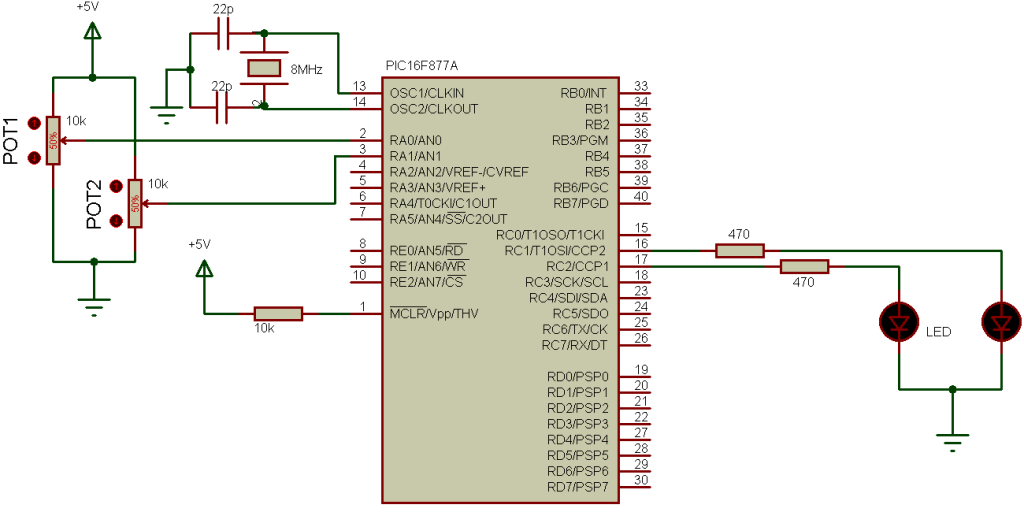# PIC16F877A PWM example with CCS PIC C compiler

The microcontroller PIC16F877A has two CCP modules and with these modules we can generate two PWM (Pulse Width Modulation) signals PWM1 related with CCP1 and PWM2 related with CCP2. Both PWM modules use Timer2 to generate signals which means the two modules will have the same frequency.
This topic shows how to use PIC16F877A PWM modules using CCS PIC C compiler.

First we have to configure the CCP module to run as a PWM using the following CCS commands:
setup_ccp1(CCP_PWM);           // Configure CCP1 as a PWM
setup_ccp2(CCP_PWM);           // Configure CCP2 as a PWM
Then we have to use Timer2 to setup the pwm frequency.
Use the following equation to compute the pwm frequency:
PWM period = [(PR2) + 1] • 4 • Tosc • (TMR2 Prescale Value)
Where PWM frequency is defined as 1/[PWM period].
Tosc = 1/(MCU_frequency)
TMR2 Prescale Value can be 1, 4 or 16.

For example for PR2 = 255 , microcontroller frequency = 8MHz and Prescale = 16 we get a PWM frequency of 488Hz.
The CCS Timer2 configuration has the following form:
setup_timer_2(mode,  period, postscale);

where: mode is TMR2 Prescale Value, period is PR2 and postscaler is not used in the determination of the PWM frequency (keep it 1).
Previous example gives the following Timer2 configuration command:
setup_timer_2(T2_DIV_BY_16, 255, 1);
The last CCS command is the PWM duty cycle command:
set_pwm1_duty(value);